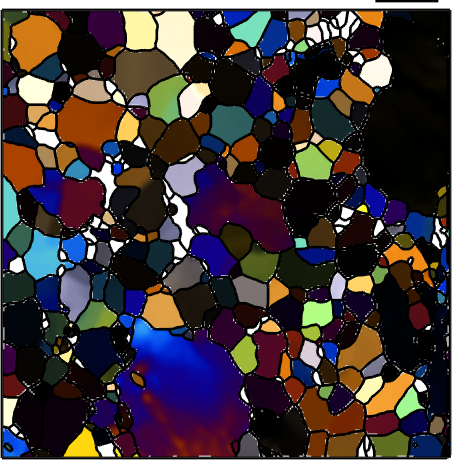Birefrigence edit page

Birefringence is the optical property of a material having a refractive index that depends on the polarization and propagation direction of light. It is one of the oldest methods to determine orientations of crystals in thin sections of rocks.

## Import Olivine Data

In order to illustarte the effect of birefringence lets consider a olivine data set.## The refractive index tensor

The refractive index of a material describes the dependence of the speed of light with respect to the propagation direction and the polarization direction. In a linear world this relation ship is modeled by a symmetric rank 2 tensor - the so called refractive index tensor, which is usually given by it principle values: n_alpha, n_beta and n_gamma. In orthorhombic minerals such as olivine the principal values are parallel to the crystallographic axes. Care has to be applied when associating the principle values with the correct axes.

For Forsterite the priniple refractive values are

with the largest refractive index n_gamma beeing alligned with the a-axis, the intermediate index n_beta with the c-axis and the smallest refractive index n_alpha with the b-axis. Hence, the refractive index tensor for Forsterite takes the form

For Fayalite the priniple refractive values

are aligned to the crystallograhic axes in an analogous way. Which leads to the refractive index tensor

The refractive index of composite materials like Olivine can now be modelled as the weighted sum of the of the refractive index tensors of Forsterite and Fayalite. Lets assume that the relative Forsterite content (atomic percentage) is sgiven my

Then is refractive index tensor becomes

## Birefringence

The birefringence describes the difference n in diffraction index between the fastest polarization direction pMax and the slowest polarization direction pMin for a given propagation direction vprop.

If the polarization direction is ommited the results are spherical functions which can be easily visualized.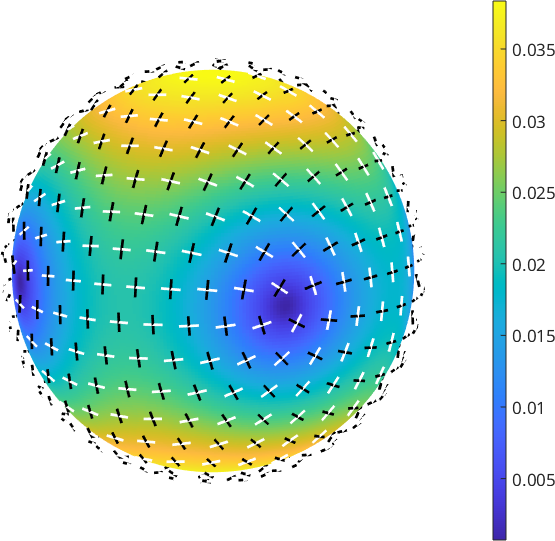## The Optical Axis

The optial axes are all directions where the birefringence is zero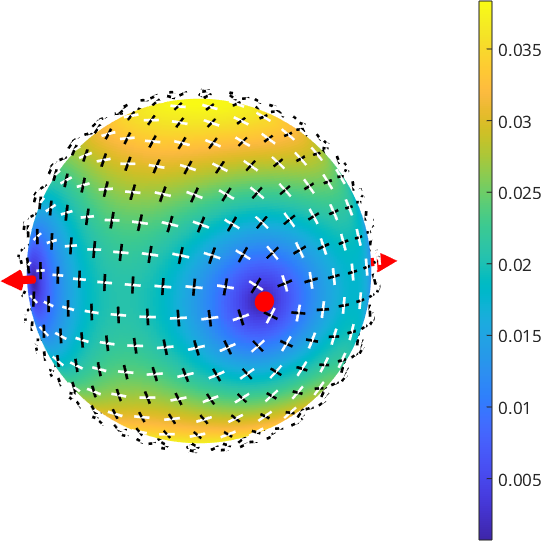## Spectral Transmission

If white light with a certain polarization is transmited though a crystal with isotropic refrative index the light changes wavelength and hence appears collored. The resulting color depending on the propagation direction, the polarization direction and the thickness can be computed by

Effectively, the rgb value depend only on the angle tau between the polariztzion direction and the slowest polarization direction pMin. Instead of the polarization direction this angle may be specified directly

If the angle tau is fixed and the propagation direction is ommited as input MTEX returns the rgb values as a spherical function. Lets plot these functions for different values of tau.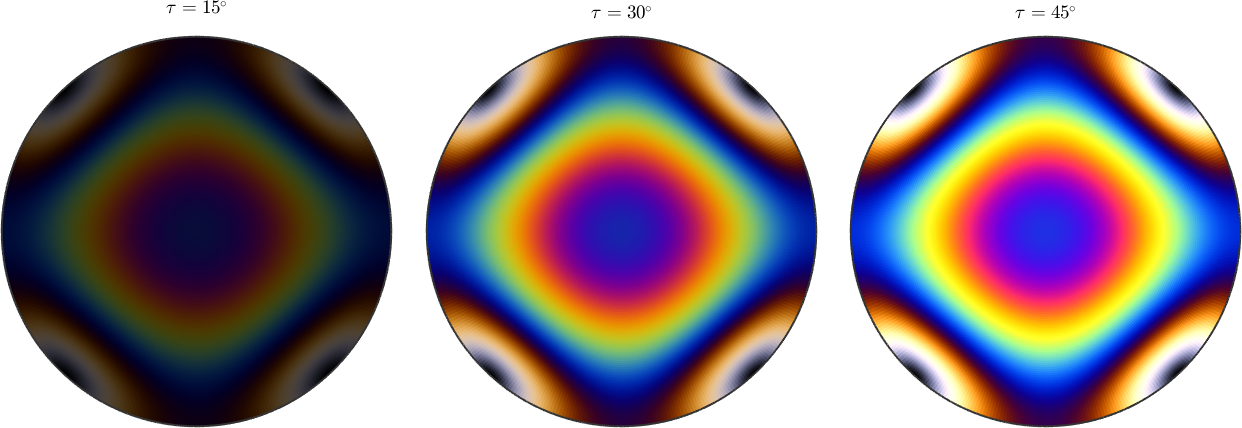Usually, the polarization direction is chosen at angle phi = 90 degree of the analyzer. The following plots demonstrate how to change this angle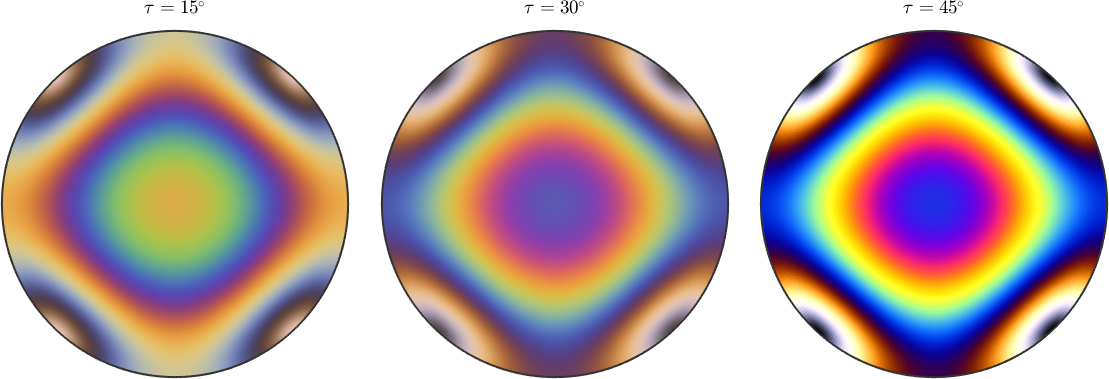## Spectral Transmission at Thin Sections

All the above computations have been performed in crystal coordinates. However, in practical applications the direction of the polarizer as well as the propagation direction are given in terms of specimen coordinates.

As usal we have two options: Either we transform the refractive index tensor into specimen coordinates or we transform the polarization direction and the propagation directions into crystal coordinates. Lets start with the first option: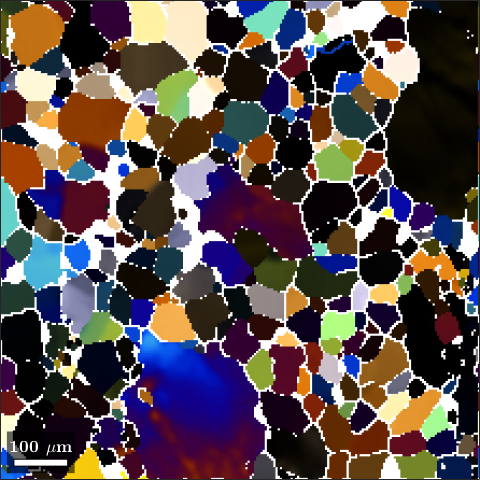and compare it with option two: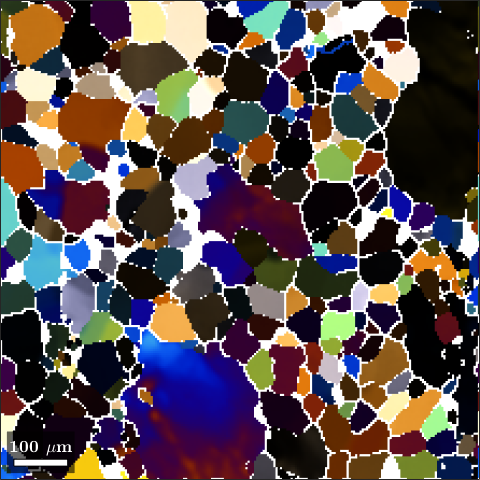## Spectral Transmission as a color key

The above computations can be automized by defining a spectral transmission color key.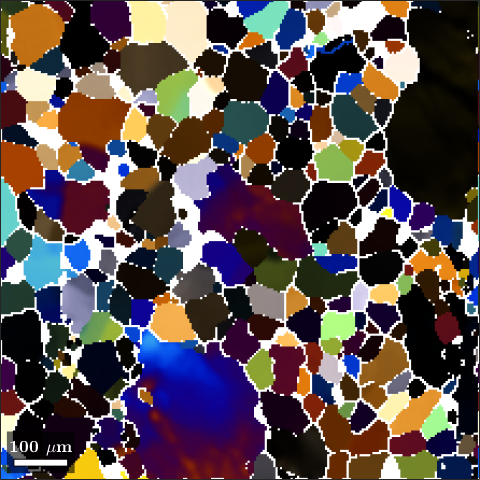As usual we me visualize the color key as a colorization of the orientation space, e.g., by plotting it in sigma sections: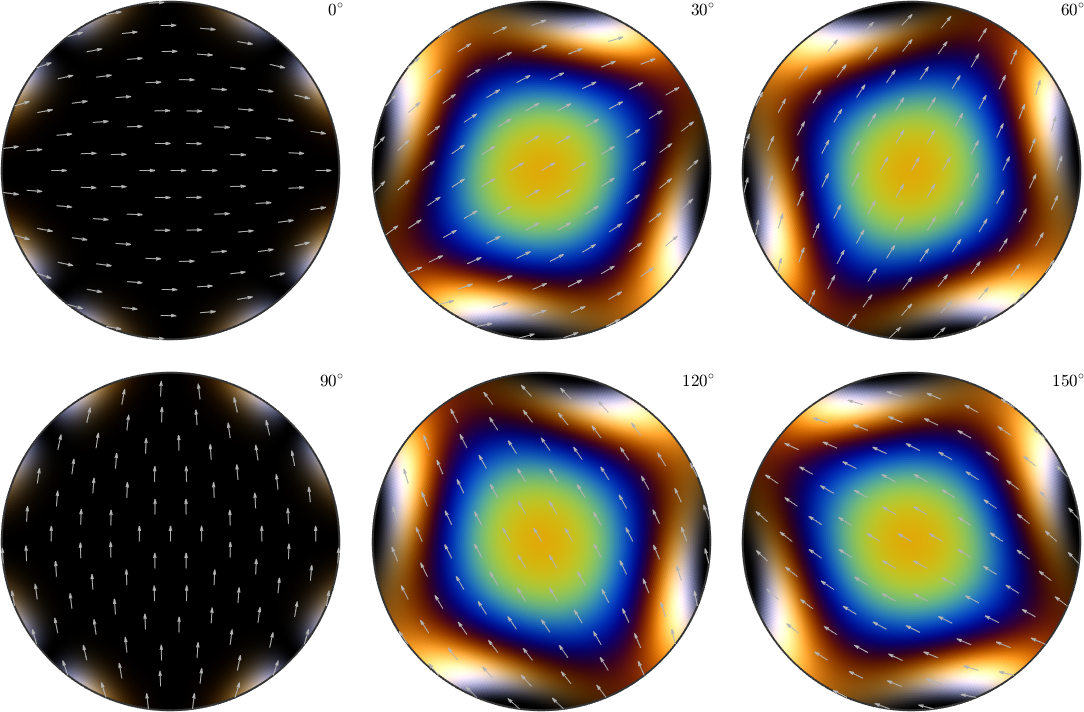## Circular Polarizer

In order to simulate we a circular polarizer we simply set the polarizer direction to empty, i.e.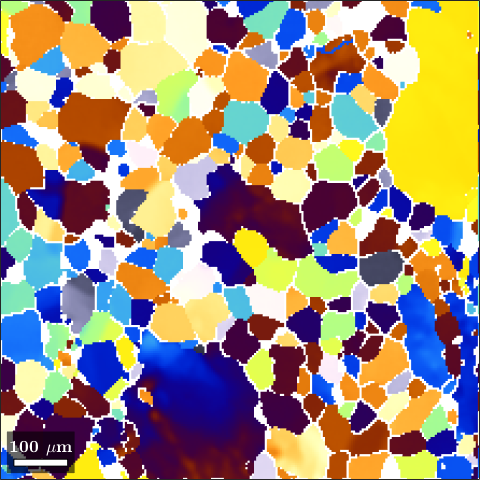## Illustrating the effect of rotating polarizer and analyser simultanously